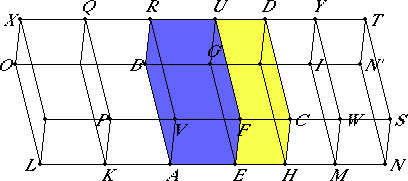# Proposition 25

If a parallelepipedal solid is cut by a plane parallel to the opposite planes, then the base is to the base as the solid is to the solid.

Let the parallelepipedal solid ABCD be cut by the plane FG which is parallel to the opposite planes RA and DH.

I say that the base AEFV is to the base FHCF as the solid ABFU is to the solid EGCD.

Produce AH in each direction. Make any number of straight lines AK and KL equal to AE, and any number HM and MN equal to EH. Complete the parallelograms LP, KV, HW, and MS and the solids LQ, KR, DM, and MT.XI.24

Then, since the straight lines LK, KA, and AE equal one another, therefore the parallelograms LP, KV, and AF equal one another, KO, KB, and AG equal one another, and further LX, KQ, and AR equal one another, for they are opposite. For the same reason the parallelograms EC, HW, and MS are equal one another, HG, HI, and IN equal one another, and further, DH, MY, NT and equal one another.

XI.Def.10

Therefore in the solids LQ, KR, and AU three planes equal three planes. But the three planes equal the three opposite, therefore the three solids LQ, KR, and AU equal one another. For the same reason the three solids ED, DM, and MT also equal one another. Therefore, the solid LU is the same multiple of the solid AU that the base LF is of the base AF. For the same reason, the solid NU is the same multiple of the solid HU that the base NF is of the base FH.

And, if the base LF equals the base NF, then the solid LU also equals the solid NU; if the base LF exceeds the base NF, then the solid LU also exceeds the solid NU; and, if one falls short, then the other falls short.

V.Def.5

Therefore, there being four magnitudes, the two bases AF and FH, and the two solids AU and UH, equimultiples have been taken of the base AF and the solid AU, namely the base LF and the solid LU, and equimultiples of the base HF and the solid HU, namely the base NF and the solid NU, and it has been proved that, if the base LF exceeds the base FN, then the solid LU also exceeds the solid NU; if the bases are equal, then the solids are equal; and if the base falls short, then the solid falls short. Therefore, the base AF is to the base FH as the solid AU is to the solid UH.

Therefore If a parallelepipedal solid is cut by a plane parallel to the opposite planes, then the base is to the base as the solid is to the solid.

Q.E.D.

## Guide

This is the first of the propositions on volumes of solids. Most of the rest of this book deals with volumes of parallelepipeds, and Book XII develops the theory of volumes for pyramids, prisms, cones, cylinders, and spheres.

Euclid’s foundations for volume are (1) his definition XI.Def.10 which says that if two solid figures have congruent faces, then the solids are equal, and (2) solids are magnitudes for which cut and paste principles hold. See the comments on XI.Def.10 for details.

#### Outline of the proof

The analogous proposition for two dimensions is proposition VI.1. In both propositions the ratio of two figures in one dimension is shown to be equal to the ratio of two figures in another dimension. In this proposition, the dimensions are 2 and 3, while in VI.1, the dimensions are 1 and 2. Eudoxus’ definition of proportion, V.Def.5, allows these ratios of different kinds to be compared.

The goal of this proof is to show that the ratio of the bases of the the two parallelepipeds is the same as the ratio of the two parallelepipeds themselves. The parallelepiped AU has the parallelogram AF as its base, while the parallelepiped HU has the parallelogram HF as its base. Thus, the goal is to derive the proportion

AU : HU = AF : HF.

By the definition of proportion, V.Def.5, that means for any number m and any number n that

m AF >=< n HF when m AU >=< n HU.

Note that Euclid takes both m and n to be 3 in his proof, just as he did in VI.1. Now m AU equals the parallelepiped LU, n HU equals the parallelepiped NU, m AF equals the parallelogram LF, and n HF equals the parallelogram NF. So what has to be shown is that

LF >=< NF when LU >=< NU.

Euclid makes no attempt to show that; he just states it as fact:

And, if the base LF equals the base NF, then the solid LU also equals the solid NU; if the base LF exceeds the base NF, then the solid LU also exceeds the solid NU; and, if one falls short, then the other falls short.
The case of equality is based directly on definition XI.Def.10, for if the bases are equal, then all the planes bounding the solids are equal, which is the definition of the solids being equal. The two cases when one base exceeds or falls short of the other implicitly depend on finding a part of one solid equal to the whole of the other solid, equality again using XI.Def.10, then concluding that one whole solid is greater than the other whole solid (C.N.5).

#### Use of this proposition

This proposition is used for the proofs of propositions XI.31, XI.32, and XI.34.